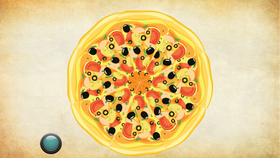« Middle School Courses
This course is included with our Coding/STEAM Curriculum - Middle School Plan# Math 201 Programming 300 Programming 201 Programming 202 Programming 301 Programming 302 JavaScript 101 Python 101 Web Development 101 Python 201 Drones 101 Augmented Reality micro:bit 101 MicroPython 101 Life Science 201 Physical Science 201 Earth Science 201 Math 201 Social Studies 201 English 201

A collection of elementary math projects that demonstrate the application of coding to solve math problems and model math concepts.

• Intermediate

## Description

Once your students have completed at least five lessons of Programming 101 or equivalent coding experience, you can assign these CCSS-aligned projects to complement your teaching on topics in geometry, measurement units, fractions, probability, and more. For example, if you’re teaching a lesson on probability, you can assign the Dice Rolls project. Your students will use coding to create a simulation of dice being rolled, then explore the probability of different outcomes by programmatically rolling a die hundreds or thousands of times.

With this collection of math projects, you can easily integrate coding and project-based learning into your curriculum. Each STEM lesson walks students through how to make a project about something they’re learning in school with step-by-step instructions. At each step, it encourages them to make their project unique and interesting, emphasizing that coding is a creative medium much like writing or drawing.

We’re constantly updating our STEM courses with new projects, so if there’s something you’d like us to add, send us a message at support@tynker.com.## What Students Learn

• Use programming for math projects
• Build a slide show on a topic
• Build a quiz game
• Make an interactive chart
• Use animation to illustrate
• Narrate using your own voice
• Use the physics engine to model
• Troubleshoot and debug programs

## Technical Requirements

* Online courses require a modern desktop computer, laptop computer, Chromebook, or Netbook with Internet access and a Chrome (29+), Firefox (30+), Safari (7+), or Edge (20+) browser. No downloads required.
* Tablet courses require an iPad (iOS 10+) with Tynker or Tynker Junior app installed and Internet access

## Lesson Summary

Create a quiz that demonstrates your knowledge of math.
##### Introduction
In this lesson, students will play a quiz game and answer questions on various math topics (i.e., factors, square roots, order of operations). Next, students will follow a step-by-step tutorial in the DIY (do-it-yourself) module to create their own version of the quiz game they just played. They will customize the background, use variables and messages to ask questions, and include animations and other effects to enhance the quiz game. Note: Variables are an important part of keeping track of a game’s state. For example, if a student wants to program their game to check if a player has finished doing something, use variables!

After completing the DIY module, students will be asked 5 quiz questions involving factors, square roots, and order of operations. By the end of the lesson, students will have experimented with coding concepts (e.g., input/output, basic math, loops, functions, events, conditionals, messaging, and visibility) while creating a math quiz game.

##### Vocabulary
• Factor: A Number that can multiply with another number to make a specified number
• Expression: Numbers, symbols, and operators that are grouped together
• Order of operations: The rule for calculating expressions, which states a specific order
• Square root of a number: A number that can be multiplied by itself to give the specified number
• Square number: A number that is multiplied by itself
• Prime number: A number that has exactly two factors
• Divisible: Can be divided without having a remainder

##### Objectives
Students will...
• Solve math problems on various topics (i.e., factors, square roots, order of operations)
• Use code blocks to create a math quiz game

##### Concepts
• conditional loops
• basic math
• input/output
• functions
• conditional wait
• simple events
• simple conditionals
• simple messaging
• visibility

##### Standards
• CCSS-Math: MP1, 6.NS.B.4, 7.NS.A.1.D, 8.EE.A.1
• CCSS-ELA: SL.6.1, SL.7.1, SL.8.1
• CSTA: 2-AP-12, 2-AP-13, 2-AP-16, 2-AP-17
• CS CA: 6-8.AP.13, 6-8.AP.16, 6-8.AP.17
• ISTE: 1.c, 1.d, 4.d, 5.c, 5.d, 6.b

## Class Presentations

These student-facing slide presentations help educators seamlessly run Tynker lessons in a virtual or physical classroom setting. Each lesson has its own set of slides that introduce the big ideas, suggest unplugged activities, and include a section for each activity module. While running lesson slides, you can switch back and forth between the activity, the slides, answer keys and other lesson materials.Lesson 1
Math Quiz
22 SlidesLesson 2
Coordinate Plane
22 SlidesLesson 3
Average Age
22 SlidesLesson 4
Positive and Negative Values
22 SlidesLesson 5
Double Negatives
25 SlidesLesson 6
Percents and Money
21 SlidesLesson 7
Market Price
22 SlidesLesson 8